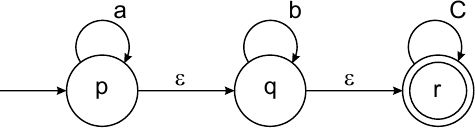MORE IN Theoretical Computer Science
MU Computer Engineering (Semester 4)
Theoretical Computer Science
December 2015
Total marks: --
Total time: --
INSTRUCTIONS
(1) Assume appropriate data and state your reasons
(2) Marks are given to the right of every question
(3) Draw neat diagrams wherever necessary

1 (a) Consider the following grammer G={V, T, P, S}, V={S, X}, T{0, 1} and productions P are
S→0| 0X1 | 01S1
X→ 0XXI| 1S
S is start symbol. Show that above grammer is ambiguous.
5 M
1 (b) State and prove the halting problem.
5 M
1 (c) Convert following ε-NFA to NFA without ε.5 M
1 (d) Prove that Language L={0n10n for n=0, 1, 2, ....} is not regular.
5 M

2 (a) Consider the following grammer G=(V, T, P, S), V={S, X, Y}, T{a, b} and productions P are
S→XYX
X→aX|ε
Y→bY|ε
Convert this grammer in Chomsky Normal Form (CNF).
10 M
2 (b) Design DPDA to acept language L={x∈{a, b}* | Na(x)>Nb(x)}, Na(x)>Nb(x) means number of a's are greater than number of b's in string x:
10 M

3 (a) Design Turing machine to accept the language L=set of string with equal number of a's and b's.
10 M
3 (b) Design the DFA to accept the language containing all the strings over ∑={a, b, c} that starts and ends with different symbols.
10 M

4 (a) Design Moore Machine for the input from (0+1+2)* which print the residue modulo 5 of the input treated as ternary number.
10 M
4 (b) State and prove pumping lemma for context free language.
10 M

5 (a) Convert the following grammer into finite automata.
S→aX | bY | a | b
X→aS | bY | b
Y→aX | bS.
5 M
5 (b) Compare recursive and recursively enumerable languages.
5 M
5 (c) State and prove Rice's theorem.
10 M

6 (a) Write regular expression for the following languages.
language containing all the string in which every pair of adjacent a's appears before any pair of adjacent b's, over the alphabet ∑{a, b}:
ii) language containing all the string in which all possible combination of a's and b's is present but strings does not have two consecutive a's, over the alphabet ∑{a, b}.
10 M
6 (b) Write short note on 'Unversal Turing Machine'.
5 M
6 (c) Explain variation and equivalences of Turing machine.
10 M

More question papers from Theoretical Computer Science